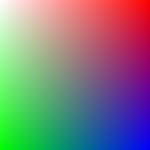## Untermenü03.10.2023 - 16:42:36

The following function generates a gradient. You can configure width, height and the colors of the 4 corners.
Sample of a gradient generated by this function:``` <?php function gradient(\$w=100, \$h=100, \$c=array('#FFFFFF','#FF0000',                             '#00FF00','#0000FF'), \$hex=true) {  /*  Generates a gradient image  Author: Christopher Kramer  Website: http://www.christosoft.de/Tutorials/Farbverlauf    Parameters:  w: width in px  h: height in px  c: color-array with 4 elements:     \$c:   top left color     \$c:   top right color     \$c:   bottom left color     \$c:   bottom right color      if \$hex is true (default), colors are hex-strings like '#FFFFFF'      (NOT '#FFF')  if \$hex is false, a color is an array of 3 elements which are the     rgb-values, e.g.:  \$c=array(0,255,255);    */    \$im=imagecreatetruecolor(\$w,\$h);    if(\$hex) {  // convert hex-values to rgb   for(\$i=0;\$i<=3;\$i++) {    \$c[\$i]=hex2rgb(\$c[\$i]);   }  }    \$rgb=\$c; // start with top left color  for(\$x=0;\$x<=\$w;\$x++) { // loop columns   for(\$y=0;\$y<=\$h;\$y++) { // loop rows    // set pixel color    \$col=imagecolorallocate(\$im,\$rgb,\$rgb,\$rgb);    imagesetpixel(\$im,\$x-1,\$y-1,\$col);    // calculate new color     for(\$i=0;\$i<=2;\$i++) {     \$rgb[\$i]=       \$c[\$i]*((\$w-\$x)*(\$h-\$y)/(\$w*\$h)) +       \$c[\$i]*(\$x     *(\$h-\$y)/(\$w*\$h)) +       \$c[\$i]*((\$w-\$x)*\$y     /(\$w*\$h)) +       \$c[\$i]*(\$x     *\$y     /(\$w*\$h));    }   }  }  return \$im; } function hex2rgb(\$hex) {  \$rgb=hexdec(substr(\$hex,1,2));  \$rgb=hexdec(substr(\$hex,3,2));  \$rgb=hexdec(substr(\$hex,5,2));  return(\$rgb); } // usage example \$image=gradient(300, 300, array('#000000', '#FFFFFF',                  '#FF0000', '#0000FF')); header('Content-type: image/png'); imagepng(\$image); imagedestroy(\$image); ?> ```

Parameters are width, height and the colors of the 4 corners. An image handle with the gradient-image will be returned.
You can directly output the image or copy it onto another image using imagecopy. This can be useful to generate a shadow or glow-effect. For a glow-effect, generate 8 gradients, one for each corner and one for each sides and place them around your image. The outer side of the gradients has the background color, the inner side has a light color such as white.

Attention: This function is not optimized for speed and might become slow when gradients are getting big. If you want to generate shadow or glow effects, caching the images is mostly possible so this should not be an issue.

Note: I strongly recommend truecolor for gradients. So if you copy the gradient onto another image, make sure it was generated as a truecolor image.

This function was originally posted by me on php.net.